Dear Aspirants,

### Quantitative Aptitude Quiz For SBI PO/Clerk Prelims

Numerical Ability or Quantitative Aptitude Section has given heebie-jeebies to the aspirants when they appear for a banking examination. As the level of every other section is only getting complexand convoluted, there is no doubt that this section, too, makes your blood run cold. The questions asked in this section are calculative and very time-consuming. But once dealt with proper strategy, speed, and accuracy, this section can get you the maximum marks in the examination. Following is the Quantitative Aptitude quiz to help you practice with the best of latest pattern questions.

Directions (1-5): For the two given equations I and II.

Q1.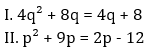if P is greater than q.
if p is smaller than q.
if p is equal to q.
if p is either equal to or greater than q.
if p is either equal to or smaller than q.

Q2.if P is greater than q.
if p is smaller than q.
if p is equal to q.
if p is either equal to or greater than q.
if p is either equal to or smaller than q.

Q3.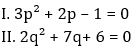if P is greater than q.
if p is smaller than q.
if p is equal to q.
if p is either equal to or greater than q.
if p is either equal to or smaller than q.

Q4.if P is greater than q.
if p is smaller than q.
if p is equal to q.
if p is either equal to or greater than q.
if p is either equal to or smaller than q.

Q5.if P is greater than q.
if p is smaller than q.
if p is equal to q.
if p is either equal to or greater than q.
if p is either equal to or smaller than q.

Directions (6-15): In each of the following questions two equations are given. You have to solve the equations and Give answer –

Q6.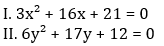if x < y
if x ≤ y
relationship between x and y cannot be determined
if x ≥ y
if x > y

Q7.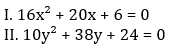if x < y
if x ≤ y
relationship between x and y cannot be determined
if x ≥ y
if x > y

Q8.if x < y
if x ≤ y
relationship between x and y cannot be determined
if x ≥ y
if x > y

Q9.if x < y
if x ≤ y
relationship between x and y cannot be determined
if x ≥ y
if x > y

Q10.if x < y
if x ≤ y
relationship between x and y cannot be determined
if x ≥ y
if x > y

Q11.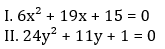if x < y
if x ≤ y
relationship between x and y cannot be determined
if x ≥ y
if x > y

Q12.if x < y
if x ≤ y
relationship between x and y cannot be determined
if x ≥ y
if x > y

Q13.if x < y
if x ≤ y
relationship between x and y cannot be determined
if x ≥ y
if x > y

Q14.if x < y
if x ≤ y
relationship between x and y cannot be determined
if x ≥ y
if x > y

Q15.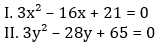if x < y
if x ≤ y
relationship between x and y cannot be determined
if x ≥ y
if x > y

You May also like to Read: